Math Olympiad Test: Area of Parallelograms and Triangles- 1

# Math Olympiad Test: Area of Parallelograms and Triangles- 1

Test Description

## 10 Questions MCQ Test Mathematics Olympiad for Class 9 | Math Olympiad Test: Area of Parallelograms and Triangles- 1

Math Olympiad Test: Area of Parallelograms and Triangles- 1 for Class 9 2022 is part of Mathematics Olympiad for Class 9 preparation. The Math Olympiad Test: Area of Parallelograms and Triangles- 1 questions and answers have been prepared according to the Class 9 exam syllabus.The Math Olympiad Test: Area of Parallelograms and Triangles- 1 MCQs are made for Class 9 2022 Exam. Find important definitions, questions, notes, meanings, examples, exercises, MCQs and online tests for Math Olympiad Test: Area of Parallelograms and Triangles- 1 below.
Solutions of Math Olympiad Test: Area of Parallelograms and Triangles- 1 questions in English are available as part of our Mathematics Olympiad for Class 9 for Class 9 & Math Olympiad Test: Area of Parallelograms and Triangles- 1 solutions in Hindi for Mathematics Olympiad for Class 9 course. Download more important topics, notes, lectures and mock test series for Class 9 Exam by signing up for free. Attempt Math Olympiad Test: Area of Parallelograms and Triangles- 1 | 10 questions in 10 minutes | Mock test for Class 9 preparation | Free important questions MCQ to study Mathematics Olympiad for Class 9 for Class 9 Exam | Download free PDF with solutions
 1 Crore+ students have signed up on EduRev. Have you?
Math Olympiad Test: Area of Parallelograms and Triangles- 1 - Question 1

### Tick the incorrect statement:

Detailed Solution for Math Olympiad Test: Area of Parallelograms and Triangles- 1 - Question 1

If the parallelograms lie on the same base and between the same parallel lines, then their areas are equal.

Math Olympiad Test: Area of Parallelograms and Triangles- 1 - Question 2

### A parallelogram and a rectangle are constructed on same base and between the same parallels, they have

Detailed Solution for Math Olympiad Test: Area of Parallelograms and Triangles- 1 - Question 2

Base = b, Distance between parallels = h
Then, Area of rectangle = area of parallelogram = bh

Math Olympiad Test: Area of Parallelograms and Triangles- 1 - Question 3

### Point O is the point of intersection of AC and BD of parallelogram ABCD and, also, PQ ∥ AD, then,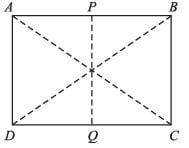Detailed Solution for Math Olympiad Test: Area of Parallelograms and Triangles- 1 - Question 3

∵ O lies in mid of the parallelogram.
∴ PQ divides parallelogram in two parts of equal areas.
∴ ar(PQDA) = 1/2 ar(ABCD)

Math Olympiad Test: Area of Parallelograms and Triangles- 1 - Question 4

P and Q are the points on AB and BC respectively of ∥gm ABCD, then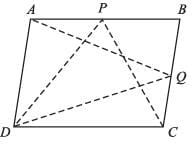Detailed Solution for Math Olympiad Test: Area of Parallelograms and Triangles- 1 - Question 4

∵ ABCD and ∆PDC lie on same base, i.e., CD and between the same parallels, i.e., AB and CD.
∴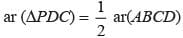Similarly,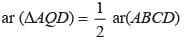⇒ ar(∆PDC) = ar(∆AQD) = 1/2 ar(quad. ABCD)

Math Olympiad Test: Area of Parallelograms and Triangles- 1 - Question 5

In ∆ABC, P, Q, R are the midpoints of sides BC, CA, AB respectively, then ar(∆PQR) =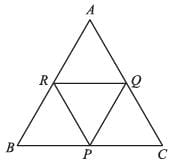Detailed Solution for Math Olympiad Test: Area of Parallelograms and Triangles- 1 - Question 5

Using midpoint theorem,
BC = 2 QR, AB = 2 PQ, AC = 2 PR.
Let the area of ∆PQR be A, then, ABC is a ∆ resulted by doubling the length of every side of ∆PQR.
∴ ar (∆ABC) = 4 ar(∆PQR) [using Heron’s formula]

Math Olympiad Test: Area of Parallelograms and Triangles- 1 - Question 6

If P, Q, R and S are midpoints of AB, BC, CD and DA of parallelogram ABCD and ar(ABCD) = 26 m2, then ar(PQRS) =

Detailed Solution for Math Olympiad Test: Area of Parallelograms and Triangles- 1 - Question 6

ar (parallelogram PQRS)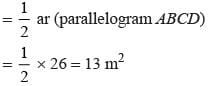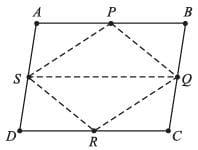Math Olympiad Test: Area of Parallelograms and Triangles- 1 - Question 7

ABC is a right angled ∆ at A, BCED, ACFG and ABMN are squares on sides ABC, AC, AB respectively. AX ⊥ DE meets BC at Y then,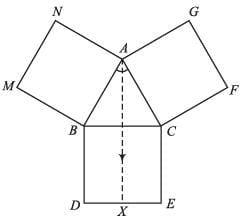Detailed Solution for Math Olympiad Test: Area of Parallelograms and Triangles- 1 - Question 7

Let AB = x, AC = y, then,
BC =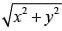(Pythagoras’ theorem)
Area (ABMN) = x2
Area (ACFG) = y2
ar(BCED) =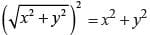∴ ar(BCED) = ar(ABMN) + ar(ACFG)

Math Olympiad Test: Area of Parallelograms and Triangles- 1 - Question 8

Ar(trap. DCYX) = K, Ar(XYBA), then K =

Detailed Solution for Math Olympiad Test: Area of Parallelograms and Triangles- 1 - Question 8

According to question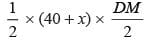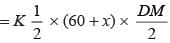⇒ (40 + 50) = K (60 + 50)
⇒ k = 9/11

Math Olympiad Test: Area of Parallelograms and Triangles- 1 - Question 9

ABCD is a trapezium in which AB ∥ DC, then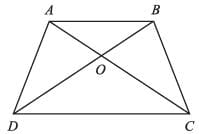Detailed Solution for Math Olympiad Test: Area of Parallelograms and Triangles- 1 - Question 9

∵ ∆ADC and ∆BDC lie on same base DC and between the same parallels, i.e., AB and DC.
∴ ar(∆ADC) = ar(∆BDC) ar(AOD) + ar(DOC) = ar(BOC) + ar(DOC) ⇒ ar(AOD) = ar(BOC).

Math Olympiad Test: Area of Parallelograms and Triangles- 1 - Question 10

ABCD is a rectangle with O as any point in its interior. If ar (∆AOD) = 3 cm2, and ar(∆BOC) = 6cm2, then ar(ABCD) =

Detailed Solution for Math Olympiad Test: Area of Parallelograms and Triangles- 1 - Question 10

Draw XY ∥ AD ∥ BC and PQ ∥ AB ∥ DC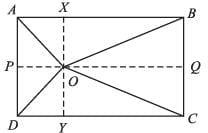Now ar(∆AOB) =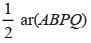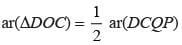⇒ ar(∆AOB) + ar(∆DOC) =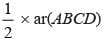⇒ ar(∆AOD) + ar(∆BOC) =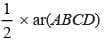⇒ ar(ABCD) = 2 × (3 + 6) = 18 cm2

## Mathematics Olympiad for Class 9

1 videos|43 tests
 Use Code STAYHOME200 and get INR 200 additional OFF Use Coupon Code
Information about Math Olympiad Test: Area of Parallelograms and Triangles- 1 Page
In this test you can find the Exam questions for Math Olympiad Test: Area of Parallelograms and Triangles- 1 solved & explained in the simplest way possible. Besides giving Questions and answers for Math Olympiad Test: Area of Parallelograms and Triangles- 1, EduRev gives you an ample number of Online tests for practice

## Mathematics Olympiad for Class 9

1 videos|43 tests